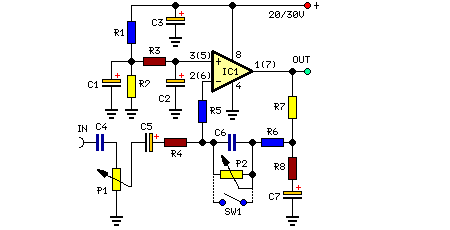# Learning Electronics

Learn to build electronic circuits

# Stereo Preamplifier With Bass Boost

High Quality, simple design, DC 20v to 30v supply

This preamplifier was designed to cope with CD players, tuners, tape recorders etc., providing an ac voltage gain of 4, in order to drive less sensitive power amplifiers. As modern Hi-Fi home equipment is frequently fitted with small loudspeaker cabinets, the bass frequency range is rather sacrificed. This circuit features also a bass-boost, in order to overcome this problem. You can use a variable resistor to set the bass-boost from 0 to a maximum of +16dB @ 30Hz. If a fixed, maximum boost value is needed, the variable resistor can be omitted and substituted by a switch.

Circuit Diagram:Large View

Parts:

P1 = 10K
P2 = 100K
R1 = 100K
R2 = 100K
R3 = 15K
R4 = 10K
R5 = 22K
R6 = 15K
R7 = 1K
R8 = 470R
C1 = 2.2uF-25v
C2 = 2.2uF-25v
C3 = 470uF-35v
C4 = 1uF-35V
C5 = 2.2uF-25v
C6 = 47nF-63v
C7 = 22uF-25v
IC1 = TL072, Opamp
SW1 = DPST Switch

Notes:
• Schematic shows left channel only, but R1, R2, R3 and C1, C2, C3 are common to both channels.
• For stereo operation P1, P2 (or SW1), R4, R5, R6, R7, R8 and C4, C5, C6, C7 must be doubled.
• Numbers in parentheses show IC1 right channel pin connections.
• A log type for P2 ensures a more linear regulation of bass-boost.
• Needing a simple boost-in boost-out operation, P2 must be omitted and SW1 added as shown in the diagram.
• For stereo operation SW1 must be a DPST type.
• Please note that, using SW1, the boost is on when the switch is open, and off when the switch is closed.

Source : www.redcircuits.com